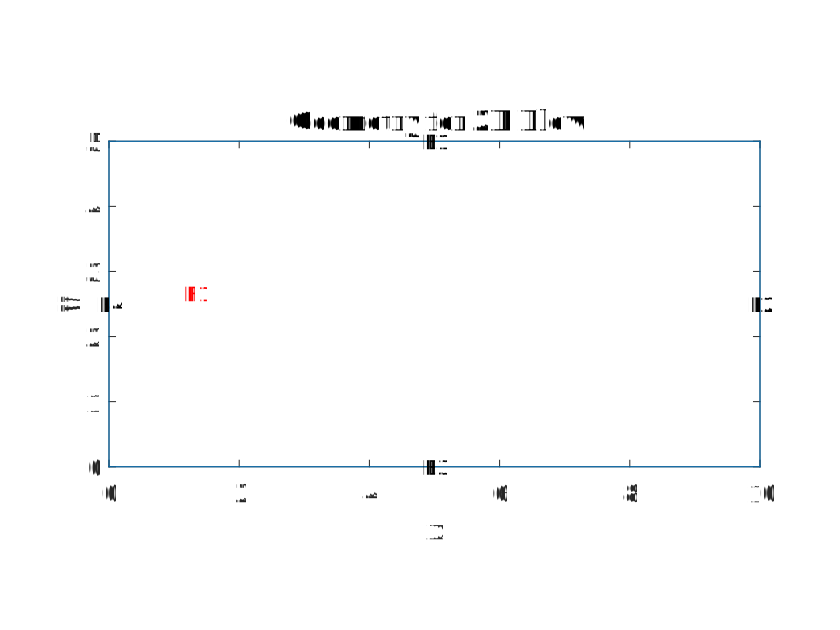# Can we solve convection diffusion equation using pdetoolbox given a is constant vector?

조회 수: 10(최근 30일)
Hashim 2022년 12월 6일
편집: Hashim 2022년 12월 7일
Hi!
We would like to solve the following 2D convection diffusion equation. Would it be possible using vanilla MATLAB pdetoolbox?whereor the flow is laminar in simpler words.
##### 댓글 수: 2표시숨기기 이전 댓글 수: 1
Hashim 2022년 12월 7일
편집: Hashim 2022년 12월 7일Hopefully I can explain my system using the domain/geometry image above. So, for my system I have a flow incoming E4 and through E2. This flow is laminar and I want it to be explained via the equation given in my original post. Now at E3 I have a heterogenous reaction at the surface of an electrode. This involves the species in the flow getting oxidized at E3 via some kinetics which I denoted by.
In this case the velocity component in the x or axial direction in the direction of y/radial is important which is why the inclusion. This is perhaps because only the flux perpendicular to the E3 is crucial to our response. Now my understanding so far is that the only the gradient across the y or radial direction is important or so suggests the literature.
Okay I have now made a little correction to the original PDE as the diffusion into y is what is important as well the convective effect on the concentrartion profile of the species of interest in the x direction. Would this possible to do in the pde toolbox? or do I need external toolboxes which I do know that I will need if my flow is turbulent.

댓글을 달려면 로그인하십시오.

### 범주

Find more on Eigenvalue Problems in Help Center and File Exchange

R2021b

### Community Treasure Hunt

Find the treasures in MATLAB Central and discover how the community can help you!

Start Hunting!